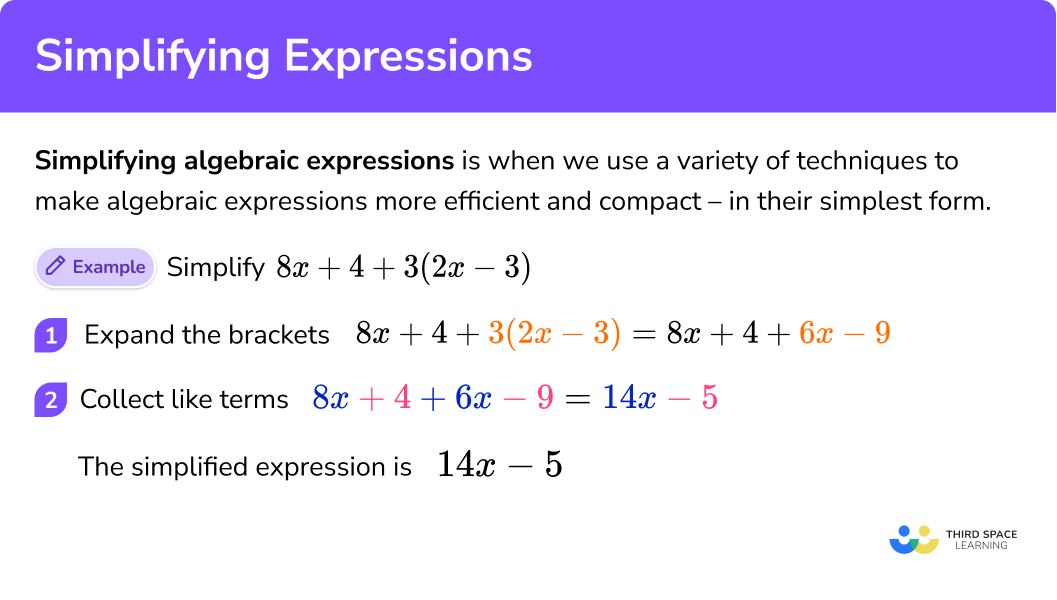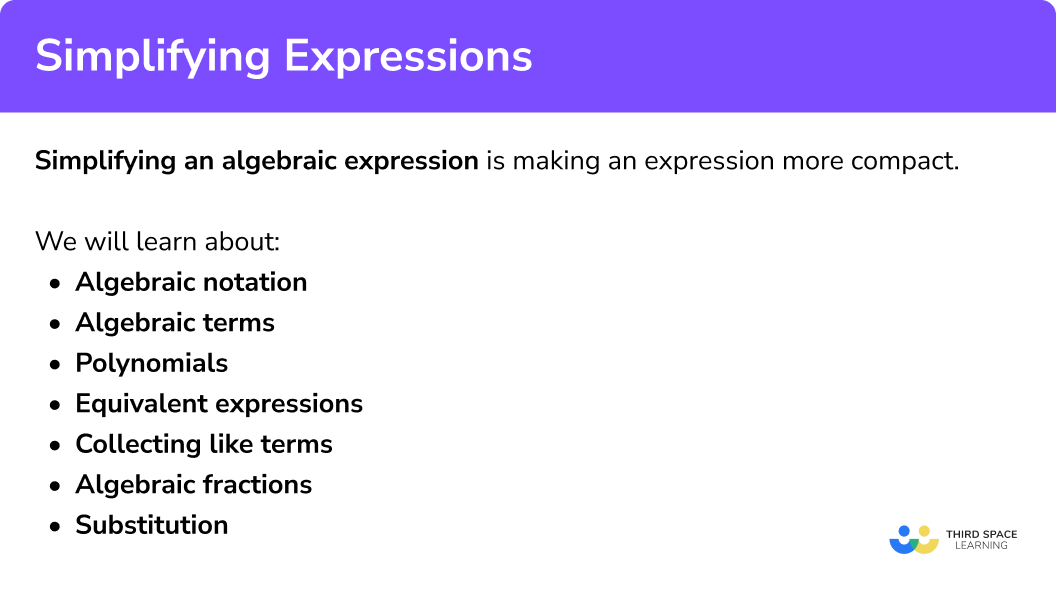# Simplifying Expressions

Here is everything you need to know about simplifying algebraic expressions for GCSE maths (Edexcel, AQA and OCR). You’ll learn how to collect like terms, write and simplify expressions, and how to simplify algebraic fractions.

Look out for the simplifying expressions worksheets with correct answers, word problems and exam questions at the end.

## What does simplifying an expression mean

Simplifying an algebraic expression is when we use a variety of techniques to make algebraic expressions more efficient and compact – in their simplest form – without changing the value of the original expression.

### What does simplifying an expression mean?## How to simplify expressions

To simplify expressions first expand any brackets, next multiply or divide any terms and use the laws of indices if necessary, then collect like terms by adding or subtracting and finally rewrite the expression.

For example to simplify

$8 x+4+3(2 x-3)$

1. Expand the brackets
$8 x+4+6 x-9$

2Collect like terms

\begin{aligned} 8 x+6 x&=14 x \\ 4-9&=-5 \end{aligned}

3Rewrite the expression

\begin{aligned}8 x+4+3(2 x-3) \\ =14 x-5\end{aligned}

### Explain how to simplify expressions## How to simplify an expression by collecting like terms

In order to simplify an algebraic expression we need to ‘collect the like terms’ by grouping together the terms that are similar:

When we highlight the like terms, we must include the sign in front of the term and where necessary identify the negative numbers.

+8x

8x

-2y

2y

## What are like terms?

Like terms have the same combination of variables and/or numbers as each other, but the coefficients could be different.

For example…

4 and 9 are like terms

3x and 5x are like terms

2ab and -5ab are like terms

BUT

8 and 3x are not like terms

4y and 2x are not like terms

x2 and x are not like terms

## Methods of simplifying expressions

### 1. Collecting like terms

Example of collecting like terms

$5 x+3 y+4-2 x+8 y-7$

1 Identify the like terms

The terms involving x are like terms. The terms involving y are like terms. The constant terms are like terms.

The plus (or minus) sign belongs to the term before it.

2 Group the like terms

$5 x-2 x+3 y+8 y+4-7$

3 Combine the like terms by adding or subtracting

\begin{aligned} 5 x-2 x=3 x \\\\ 3 y+8 y=11 y \\\\ 4-7=-3 \end{aligned}

So,

\begin{aligned} 5 x+3 y+4-2 x+8 y-7 \\\\ =3 x+11 y-3 \end{aligned}

Step-by-step guide: Collecting like terms

### 2. Multiplying and dividing algebra

Example of multiplying and dividing algebra

$\frac{3 a b \times 4 a c}{2 a}$

1 Simplify the numerator

$3 a b \times 4 a c=12 a^{2} b c$

2 Divide by the denominator

$12 a^{2} b c \div 2 a=6 a b c$

So,

$\frac{3 a b \times 4 a c}{2 a}=6 a b c$

### 3. Expanding brackets

Example of expanding brackets:

$3(2 x+5)$

1 Multiply the term outside of the bracket by the first term inside the bracket

$3 \times 2 x=6 x$

2 Multiply the term outside the bracket by the second term inside the bracket.

$3 \times 5=15$

So,

$3(2 x+5)=6 x+15$

Step-by-step guide: Expanding brackets

### 4. Algebraic fractions

Example of algebraic fractions

Simplify

$\frac{12xy}{8x}$

1 Find the highest common factor (HCF) of the numerator and denominator.

The HCF of 12xy and 8x is 4x

2 Divide the numerator and the denominator by this value.

Numerator

$12xy \div 4x=3y$

Denominator

$8x\div 4x=2$

3 Rewrite the simplified fraction

$\frac{12xy}{8x}$

$=\frac{3y}{2}$

Step-by-step guide: Algebraic fractions

### 5. Write and simplify algebraic expressions

We can write algebraic expressions to help simplify problems. We will often be able to make a linear equation or a quadratic equation and solve it.

Example of writing and simplifying expressions

Write an expression for the perimeter of the shape.

1. Read the question carefully and highlight the key information.

Key words:

Expression: a set of terms that are combined using (+, −, ✕ and ÷)

Perimeter: the distance around the edge of a shape

We need to add together each of the lengths of the shape.

2Write an expression and simplify.

We then simplify the following expression by adding and subtracting the terms.

\begin{aligned} Perimeter&=\color{#00BC89}{2x}\color{#7C4DFF}{+3}\color{#00BC89}{+x}\color{#7C4DFF}{-2}\color{#00BC89}{+2x}\color{#7C4DFF}{+3}\color{#00BC89}{+x}\color{#7C4DFF}{-2}\\ &=\color{#00BC89}{6x}\color{#7C4DFF}{+2} \end{aligned}

## Examples of simplifying expressions

### Example 1: collecting like terms with one variable and one constant

Simplify

$8x+5-2x+6$

1. Underline the similar terms in the expression and combine them.

\begin{aligned}8 x-2 x=6 x \\\\\ 5+6=11 \end{aligned}

2Rewrite the expression.

\begin{aligned} 8 x+5-2 x+6 \\\\ =6 x+11 \end{aligned}

### Example 2: collecting like terms with multiple variables and one constant

Simplify

$5xy+3y-4-2xy-8y+7$

\begin{aligned} 5 x y-2 x y=3 x y \\\\ 3 y-8 y=-5 y \\\\ -4+7=3 \end{aligned}

\begin{aligned} 5 x y+3 y-4-2 x y-8 y+7 \\\\ =3 x y-5 y+3 \end{aligned}

### Example 3: expanding brackets

Expand:

$3 x\left(3-2 y+5 x^{2}\right)$

$3 x \times 3 = 9 x$

$3 x \times-2 y=-6 x y$

$3 x \times 5 x^{2}=15 x^{3}$

So,

\begin{aligned} 3 x\left(3-2 y+5 x^{2}\right) \\\\ =9 x-6 x y+15 x^{3} \end{aligned}

### Example 4: algebraic fractions

Simplify

$\frac{9x^{2}y}{15x^{3}}$

The HCF of 9 x2 y and 15x3 is

$3 x^{2}$

Numerator

$9 x^{2} y \div 3 x^{2}=3 y$

Denominator

$15 x^{3} \div 3 x^{2}=5 x$

$\frac{9x^{2}y}{15x^{3}}$

$=\frac{3y}{5x}$

### Example 5: algebraic fractions

Simplify

$\frac{8x^{3}-6xy}{4x^{2}y}$

It is easier to find the HCF for this example if we factorise the numerator.

8x3 6xy can be written as 2x(4x2 3y).

It is now easier to see that the HCF of 8x3 6xy and 4x2y is 2x

Numerator

$\left(8 x^{3}-6 x y\right) \div 2 x=4 x^{2}-3 y$

We have to divide both terms by 2x

Denominator

$4 x^{2} y \div 2 x=2 x y$

$\frac{8x^{3}-6xy}{4x^{2}y}$

$=\frac{4x^{2}-3y}{2xy}$

### Example 6: algebraic fractions

Simplify

$=\frac{x^{2}-2x-15}{x^{2}-9}$

We will need to factorise quadratics to simplify this algebraic fraction

Numerator

$x^{2}-2 x-15=(x+3)(x-5)$

Denominator

$x^{2}-9=(x+3)(x-3)$

$=\frac{\color{#FF0C3E}{(x+3)}\color{#62F030}{(x-5)}}{\color{#FF0C3E}{(x+3)}\color{#92009E}{(x-3)}}$

$\frac{x^{2}-2 x-15}{x^{2}-9}$

$=\frac{x-5}{x-3}$

Step-by-step guide: Difference of two squares

### Example 7: expression for area

Write an expression for the area of the shape.

Key words:

Expression: a set of terms that are combined using (+, −, ✕ and ÷)

Area: the 2D space inside a shape.

This shape is a triangle. We know the formula to find the area of a triangle is:

$\text { Area of triangle }=\frac{\text { base } \times \text { height }}{2}$

We need to multiply the base and height of the shape then divide by 2.

$\frac{(2x+2)(3x+2)}{2}$

$= \frac{6x^{2}+10x+4}{2}$

$=3x^{2}+5x+2$

### Example 8: worded problem

Sophie is x years old,

Emily is three years younger than Sophie

Ameila is four  times older than Sophie.

Write an expression for each of their ages.

We are told that Sophie is x years old

Emily is three years younger than Sophie, so three less than x is x − 3

Ameila is four times older than Sophie, so four lots of x − 3 is 4(x − 3).

We need brackets because we are multiplying all of x − 3 by 4

Sophie is x years old

Emily is x − 3 years old

Ameila is 4(x − 3) = 4x − 12 years old

### Common misconceptions

• The sign in front of the term is part of it

When we underline the like terms, we must include the sign in front of the

+8x\qquad +8x

-2y\qquad -2y

• Terms with a coefficient of 1

For terms with a coefficient of 1 we don’t need to write the 1

\begin{aligned}
1x&=x\\
1ab&=ab\\
1y^{2}&=y^{2}\\
\end{aligned}

• The commutative property

When adding and multiplying, the order in which we calculate doesn’t matter

2x+3x=3x+2x=5x



and

2a3b=3b2a=6ab

This is not the case for subtracting and dividing.

• Using exponents (powers)

In order for two terms to be ‘like terms’ they need the same combination of variables.

3x2and 5x2are like terms

2a2b and -5a2bare like terms

BUT

3x2and 5x are not like terms

2a2b and -5ab are not like terms

• Using brackets (parentheses)

When multiplying an expression by a value we need to use brackets so that each term is multiplied.

2(y+4)\\
2\times y+4


### Simplifying expressions practice questions

1. Simplify

7+2a-9+6a

2+8a-2-4a-2+8a6aFor the constant terms, we have

7-9=-2

For the variable terms, we have

2a+6a=8a

This means

7+2a-9+6a=-2+8a

2. Simplify

8ab-8a-7ab-3a

ab-5a15ab-11aab-11a4aBy considering like terms, we have 8ab-7ab=ab and -8a-3a=-11a .

This means 8ab-8a-7ab-3a=ab-11a .

3. Simplify

-2xy+3x^{2}y+7x+5x^{2}y-6xy

4xy+8x^{2}y+7x-8xy-8x^{2}y-7x-8xy+8x^{2}y+7x7x^{2}yBy considering like terms, we have -2xy-6xy=-8xy and 3x^{2}y+5x^{2}y=8x^{2}y and 7x .

This means -2xy+3x^{2}y+7x+5x^{2}y-6xy=-8xy+8x^{2}y+7x .

4a. Write an expression for the perimeter of the shape:6x+144x^{2}+22x+308x+224x+11By working out the missing side lengths as algebraic expressions, and adding together all side lengths we have:

Perimeter =2 x+5+x+1+x+3+x+5+x+2+2 x+6 =8 x+22

4b. Write an expression for the area of the shape:4x^{2}+22x+303x^{2}+14x+156x+14x^{2}+14x+15The shape can be split into rectangles in more than one way

(2x+5)(x+1)=2x^{2}+7x+5 (x+5)(x+2)=x^{2}+7x+10 Area=3x^{2}+14x+15

OR

(x+1)(x+3)=x^{2}+4x+3 (2x+6)(x+2)=x^{2}+10x+12 Area=3x^{2}+14x+15

5. Steve is x years old.

Rachel is 11 years older than Steve.

Barry is twice as old as Rachel.

Write an expression for the total ages of Steve, Rachel and Barry.

4x+332x+113x+112(x+11)Steve’s age = x

Rachel’s age = x+11

Barry’s age = 2(x+11)=2x+22

Total ages =x+x+11+2x+22 \\
& =4x+33
\end{aligned} [/katex]

6. Simplify:

\frac{18ab}{12}

\frac{3ab}{2}3ab\frac{3}{2}\frac{6ab}{4}The highest common factor of the numerator and denominator is 6 , so we divide numerator and denominator by 6 , resulting in the simplified fraction.

7. Simplify:

\frac{12ab^{3}}{8a^{2}b}

\frac{3ab}{2}\frac{3b^{2}}{2a}\frac{3}{2}\frac{6ab}{4}The highest common factor of the numerator and denominator is 4ab , so we divide numerator and denominator by 4ab , resulting in the simplified fraction.

8. Simplify:

\frac{9a^{2}-6ab}{15ab^{2}}

\frac{3a}{15ab^{2}}\frac{3a-2b}{5b^{2}}\frac{3a(3a-2b)}{15ab^{2}}\frac{3a^{2}-2ab}{5ab^{2}}The numerator can be factorised, giving \frac{3a(3a-2b)}{15ab^{2}} after which the numerator and denominator can be divided by the highest common factor of 3a , resulting in the simplified fraction.

9. Simplify:

\frac{x^{2}+3x+2}{2x^{2}+x-1}

\frac{x+2}{2x-1}\frac{x+1}{2x-1}\frac{x+2}{x+1}\frac{x^{2}+2}{2x-1}We can factorise the numerator and denominator into double brackets, giving

\frac{(x+1)(x+2)}{(x+1)(2x-1)} and then cancel the common bracket from numerator and denominator,

which results in the simplified fraction.

10. Expand:

3 y(4+2 y-4 x)

12 y+6 y^{2}-12 x y12 y+6 y-12 x y12 y+5 y^{2}-12 x y12+6 y^{2}+12 x yWith a single bracket expansion, we must be sure to multiply each term inside the bracket by the number in front of the bracket. Make sure to include the correct index numbers.

## Simplifying expressions GCSE questions

1. Simplify: 4f – 2e + 3f + 5e

7f + 3g

(2 marks)

2. Expand and simplify: 4a(a + b) – 2(a2 – 2b)

2a2 + 4ab + 4b

(2 marks)

4. Expand and simplify:

$\frac{2x^{2}+7x-4}{x^{2}+2x-8}$

$\frac{2x-1}{x-2}$

(3 marks)

## Learning checklist

• Simplify and manipulate algebraic expressions to maintain equivalence by taking out common factors.
• Model situations or procedures by translating them into algebraic expressions.
• Simplify and manipulate algebraic expressions and algebraic fractions.
• Translate simple situations or procedures into algebraic expressions.

## Still stuck?

Prepare your KS4 students for maths GCSEs success with Third Space Learning. Weekly online one to one GCSE maths revision lessons delivered by expert maths tutors.

Find out more about our GCSE maths tuition programme.